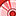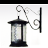# QlikView App Dev

Discussion Board for collaboration related to QlikView App Development.

Announcements
Action-Packed Learning Awaits! QlikWorld 2023. April 17 - 20 in Las Vegas: REGISTER NOW
cancel
Showing results for
Did you mean:Contributor III

Dear All,

I am trying to find the YTD in my application but when i write below expression for YTD ...I am getting nothing in my chart...getting 0 values everytime

Expression is as follows....

=

Sum( {< Date_Num={" >= \$(=Num(YearStart(Max(Date_Num)))) <= \$(=Today()-1) "} >} SALARY

or

=Sum( {< START_DATE={" >= \$(=Date(YearStart(Max(START_DATE))),'DD-MM-YYYY') <= \$(=Date(Today()-1,'DD-MM-YYYY')) "} >} SALARY)

attached my QVW also for your reference.....

Regards

Sarfaraz

1 Solution

Accepted SolutionsPartner - Champion III

Hi

=Sum({<Date_Num={">=\$(=Num(Max(YearStart(Date_Num)))) <=\$(=Num(Today()))"}>} SALARY)

or

=Sum({<Date_Num={">=\$(=Num(YearStart(Max(Date_Num)))) <=\$(=Today()-1)"}>} SALARY)

You have embedded spaces into the search expression which is causing a problem. The above two expressions work

HTH

Jonathan

Logic will get you from a to b. Imagination will take you everywhere. - A Einstein
5 RepliesMVP

Try like:

=Sum({<Date_Num={">=\$(=Num(YearStart(Max(Date_Num)))) <=\$(=(Max(Date_Num)))"}  > }   SALARY)Partner - Champion III

Hi

=Sum({<Date_Num={">=\$(=Num(Max(YearStart(Date_Num)))) <=\$(=Num(Today()))"}>} SALARY)

or

=Sum({<Date_Num={">=\$(=Num(YearStart(Max(Date_Num)))) <=\$(=Today()-1)"}>} SALARY)

You have embedded spaces into the search expression which is causing a problem. The above two expressions work

HTH

Jonathan

Logic will get you from a to b. Imagination will take you everywhere. - A EinsteinCreator II

Use the following:-

=Sum({<START_DATE={">= \$(=Date(YearStart(Max(START_DATE))),'DD-MM-YYYY')<=\$(=Date(Today()-1,'DD-MM-YYYY'))"}>}SALARY)

Your syntax was correct, but had spaces before and after a few fields, thus showing 0.

Regards

DhruvCreator III

Variables:

vStartdate: =Floor(YearStart(Max(Date_Num))

vToday =Floor((Today)-1)

Expression:

Sum( {< Date_Num={" >= \$(=vStartdate) <= \$(=vToday "} >} SALARY)Contributor III
Author

Dear Jonathan/Tresesco

Thanks for your reply !!!! now it is working as expected ...........

Regards

SarfarazCommunity Browser